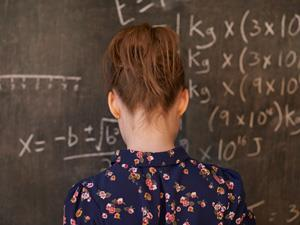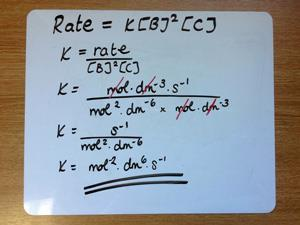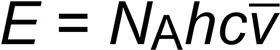What's in a number? Jonathan Agger and Kristy Turner show you how to clear up confusion with unitsEach year, faced with a group of new first-year undergraduate students in our measurements lab, we ask this question: If you were stopped by someone outside on Oxford Road (at the University of Manchester) and they asked the distance to Fallowfield, what would you reply?

A typical response might be two miles. But what would the enquirer’s reaction be if you were simply to reply ‘two’?

Everybody uses commonplace units, like miles, without a moment’s thought – we need our students to do the same with joules, pascals and watts.

### Quantity calculus

Most of our students will have met the concept of quantity calculus before, although they may not have given it a title. For example, A-level questions commonly ask students to determine the units for rate constants and equilibrium constants.In undergraduate teaching, we take this a stage further by showing our students that all compound units may be deconstructed and rewritten in terms of the seven base units of the SI system. For example, a watt is equivalent to a kg m2 s–3.

In all scientific formulas unit balance is every bit as vital as numerical balance. Take the ideal gas equation as an example:

p V = n R T

Pa × m3 = mol × J K–1 mol–1 × K

N m–2 × m3 = mol × J K–1 mol–1 × K

kg m s–2 m–2 × m3 = kg m2 s–2

kg m2 s–2 = kg m2 s–2

### Learning equations

For students, the ability to deconstruct compound units into their base SI units will aid them with verification. If, for example, they cannot remember whether a symbol goes on the top or bottom of an equation, a verification of the unit balance of the equation should elucidate the answer. Also, if they struggle to recall a particular symbol in an equation then a verification of unit balance can assist them.

The particular example we use is a formula from physics to calculate capillary rise. The students use verification of unit balance to determine the units of an unknown symbol in the equation as kg m–3 leading to the identity of the unknown symbol as the fluid density.

### Units in calculations

But the true goal of teaching students about units is to improve their ability to calculate correctly. To this end, the inclusion of units in calculations is extremely important. Almost all students will admit to ignoring units in the bulk of their calculation and simply tacking the correct unit onto the answer at the end. This disregard for units leads to trivial errors in calculation on an alarmingly frequent basis.

As an example, consider the calculation of the molar energy of the 1730 cm–1 carbonyl stretch in the infrared spectrum of methanal. Conversion of wavenumber to molar energy requires multiplication by the Planck constant, h, the speed of light, c, and the Avogadro number, NA, so:E = 6.0221 × 1023 × 6.626 × 10–34 × 2.998 × 108 × 1730 = 207 J mol–1

Unfortunately, despite correct multiplication of the values, the answer is one hundred times too small. And without a rough expectation of the correct value, the student is ignorant of the error. Including units in the calculation itself provides a major clue as to the origin of the error:

E = 6.0221 × 1023 mol–1 × 6.626 × 10–34 J s × 2.998 × 108 m s–1 × 1730 cm–1

Now, it is apparent that the s of the Planck constant cancels the s–1 of the speed of light and the m of the speed of light is supposed to cancel the cm–1 of the wavenumber, leaving the correct units for the answer of J mol–1. However, m doesn’t cancel cm–1. The error is suddenly obvious.

### Helping A-level students

In our experience, students continue to ignore units within calculations even when warned that it leads to errors. The new A-levels bring with them a greater emphasis on the mathematics (20% of examined A-level chemistry content will use applied mathematics of at least GCSE higher tier level). Perhaps greater emphasis on the units in calculation from A-level teachers might help to redress this.

Jonathan Agger is a lecturer in physical chemistry and deputy director of outreach at the University of Manchester, UK

Kristy Turner is a teacher and teacher fellow, splitting her time between Bolton School Boys’ Division and the University of Manchester, UK